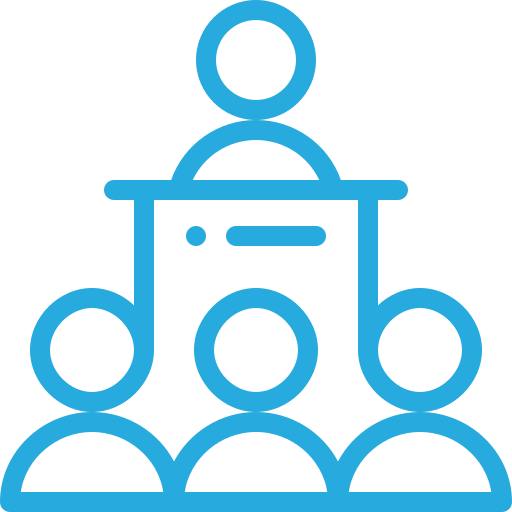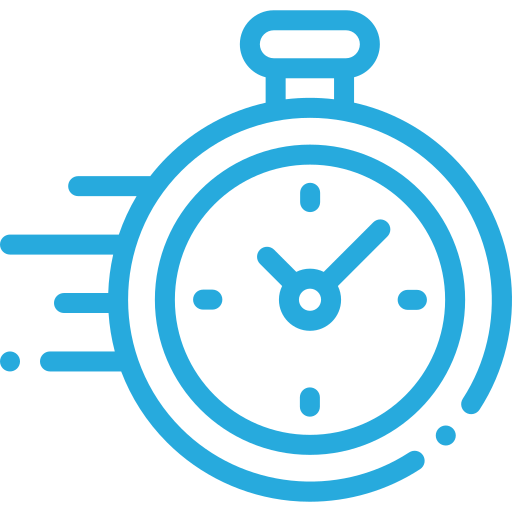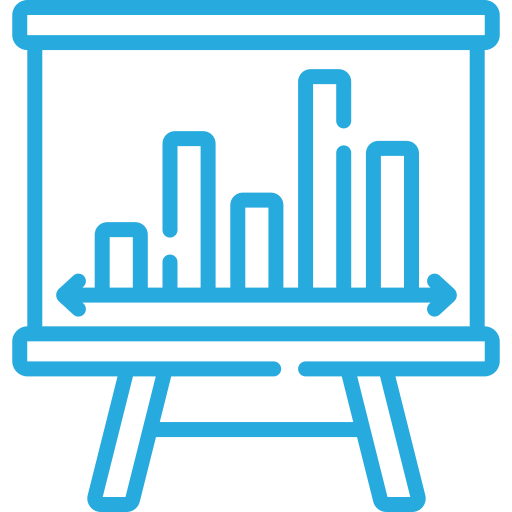MatLab

MATLAB, short for MATrix LABoratory is a programming package specifically designed for quick and easy scientific calculations and I/O. It is also a programming and numeric computing platform used by millions of engineers and scientists to analyze data, develop algorithms, and create models.#### 40

Lectures#### 50 Hours

Duration#### Intermediate

Skill level

MATLAB, short for MATrix LABoratory is a programming package specifically designed for quick and easy scientific calculations and I/O.  This course teaches computer programming to those with little to no previous experience. It uses the programming system and language called MATLAB to do so because it is easy to learn, versatile, and very useful for engineers and other professionals.  The design of the language makes it possible to write a powerful program in a few lines. The problems may be relatively complex, while these programs that solve them are relatively simple: relative, that is, to the equivalent program written in a general-purpose language, such as C++ or Java.

Students of mechanical and related streams, who successfully complete this course will become familiar with general concepts in this tool with the user interface, entering commands, and working with features such as MATLAB graphics, functions with different variables, matrix and vector operations, Symbolics, file I/O, and so on. With loads of examples and exercises, you will master MATLAB in basics and use it for complex computational processes in the field of Mechanical and related streams.

#### Introduction

• Introduction to Matlab
• Working with the MATLAB user interface

#### Basic operations and variables

• Create variables
• Save and retrieve variables
• Manipulate Variables
• Symbolic Variables
• Workspace variables

#### Math functions

• Arithmetic operations
• Simple equations
• Trigonometric functions

#### Vectors/Arrays

• Creation
• Colon operations
• Sort & Random creation
• Performing calculations with vectors
• Accessing and modifying values in vectors
• Exercise Problems

#### Matrix

• Creating matrices
• Frequently used function
• Performing calculations with matrices
• Manipulating matrixes
• Calculating statistics with matrix data
• Visualizing matrix data

#### Importing data (Table)

• Storing data as a table
• Operating on tables
• Extracting data from tables
• Modifying tables

#### Conditional Operations

• Logical operations and variables
• Finding and counting
• Logical indexing

#### Looping system

• Conditional statements
• For Loop
• DO-while Loop

#### Plots

• Line Plots
• 2D plot Modification options
• Bar Plots
• Area Plots
• 3D surface plot
• 3D Map plot
• 3D Counter Plot

#### Equational functions

• Polynomial Function
• System of the equilibrium equation
• Transcendental equation
• Tridiagonal equation

#### Differential equations

• Numerical integration
• Numerical differentiation
• Ordinary Differential equation

#### FUNCTIONS

• Creating functions
• Calling functions

#### Import and export data

• Simple equations

### Enroll Now

#### Basic

3200 /4 Months
3500
• Mode Of Delivery e-Course
• Certification On Completion
• One Time Payment
• Course Content: Available
• Email Clarification (Twice A Week)
• Non-Convertible
• Direct Interaction: Online (Weekly Once)
• Hands-On: Real-Time Projects
• Contact For Group Enrollments

#### Comfort

4999 /8 Months
5500
• Mode Of Delivery e-course
• Certification On Completion
• Course Content: Available
• One Time Payment
• Email Clarification (Twice A Week)
• Student Community
• Direct Interaction: Online (Weekly Once)
• Non-Convertible
• Hands-On: Real-Time Projects
•  Contact For Group Enrollments